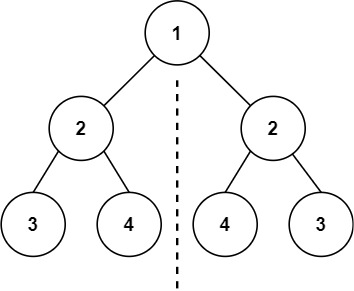## 基本概念 #

### 高度和深度 #

• 树的高度：节点到叶子节点的最大值就是其高度。
• 树的深度：高度和深度是相反的，高度是从下往上数，深度是从上往下。

var maxDepth = function(root) {
if (!root) return 0
return Math.max(maxDepth(root.left), maxDepth(root.right)) + 1
};


### 一些特性 #

1. 在二叉树的第 i 层上至多有 $2 ^ { i-1 }$ 个结点（i>=1）
2. 深度为 k 的二叉树最多有 $2^{k}-1$ 个结点（k>=1）。
3. 如果树有 n 个节点，则有 n-1 条边
4. 如果有 a 个叶节点，则有 a-1 个非叶节点
5. 具有 n 个节点的树最小深度为 $log_2n+1$

### JavaScript #

function TreeNode(val, left, right) {
this.val = (val===undefined ? 0 : val)
this.left = (left===undefined ? null : left)
this.right = (right===undefined ? null : right)
}


### Golang #

type TreeNode struct {
Val   int
Left  *TreeNode
Right *TreeNode
}


## 数据结构 #

### 对称二叉树 #101. 对称二叉树

### 二叉搜索树 #

1. 左子树的所有节点值小于根的节点值（注意不含等号）
2. 右子树的所有节点值大于根的节点值（注意不含等号）
3. 没有键值相等的节点

1. 二叉搜索树的中序遍历结果是一个有序列表，这个性质很有用。

2. 但是二叉搜索树不一定平衡，因此引申出平衡二叉搜索树。

### 平衡二叉树（AVL 树） #

1. 除了空树，任何一个结点的左子树与右子树都是平衡二叉树，并且高度之差的绝对值不超过 1。
2. 平衡二叉树在插入新的子节点时，可能会形成最小失衡子树
3. 平衡二叉树的失衡调整主要是通过旋转最小失衡子树来实现的。根据旋转的方向有两种处理方式，左旋右旋

110. 平衡二叉树

## 二叉树遍历 #

### 深度优先搜索（DFS） #

void traverse(TreeNode root) {
if (root == null) {
return;
}
// 前序位置
traverse(root.left);
// 中序位置
traverse(root.right);
// 后序位置
}


#### 先序遍历 #

144. 二叉树的前序遍历

var preorderTraversal = function(root) {
let stack = []
let ret = []

while (root || stack.length) {
while (root) {
ret.push(root.val)
stack.push(root)
root = root.left
}
root = stack.pop()
root = root.right
}
return ret
};


#### 中序遍历 #

94. 二叉树的中序遍历

var inorderTraversal = function(root) {
const res = [];
const stk = [];
while (root || stk.length) {
while (root) {
stk.push(root);
root = root.left;
}
root = stk.pop();
res.push(root.val);
root = root.right;
}
return res;
};


145. 二叉树的后序遍历

#### 反向构建 #

105. 从前序与中序遍历序列构造二叉树

106. 从中序与后序遍历序列构造二叉树

889. 根据前序和后序遍历构造二叉树

### 层次遍历 #

#### BFS 迭代方案 #

var levelOrder = function(root) {
let ret = []
if (!root) {
return ret
}
let q = []
q.push(root)
while (q.length) {
let level = q.length
ret.push([])
for (let i = 1; i <= level; i++) {
let node = q.shift()
ret[ret.length - 1].push(node.val)
if (node.left) q.push(node.left)
if (node.right) q.push(node.right)
}
}
return ret
};


#### DFS 递归方案 #

DFS 递归方案比较常规，需要一个全局的数组和一个表示层级的 level 索引：

var levelOrder = function(root) {
let ret = []
let helper = function(root, level) {
if (!root) return
if (ret.length === level) ret.push([])
ret[level].push(root.val) // 这里是先序，改成中序、后序都可以。
helper(root.left, level + 1)
helper(root.right, level + 1)
}
helper(root, 0)
return ret
};


## 常见操作 #

### 翻转二叉树 #

226. 翻转二叉树 考虑递归实现。

var invertTree = function(root) {
if (!root) {
return root
}
let left = invertTree(root.left)
let right = invertTree(root.right)

root.left = right
root.right = left
return root
};


## 其他题型 #

### 公共祖先问题（LCA） #

var lowestCommonAncestor = function (root, p, q) {
let find = function (root, p, q) {
if (root === null) return null;
if (root === p || root === q) return root;
let left = find(root.left, p, q);
let right = find(root.right, p, q);
if (left !== null && right !== null) return root;
return left !== null ? left : right;
};
find(root, p, q);
};


### todo #

589. N 叉树的前序遍历（熟悉 N 叉树）

662. 二叉树最大宽度 （请分别使用 BFS 和 DFS 解决，空间复杂度尽可能低）

834. 树中距离之和

967. 连续差相同的数字 （隐形树的遍历）

1145. 二叉树着色游戏（树上进行决策）